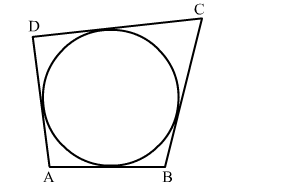# In the adjoining figure, a circle touches all the four sides of a quadrilateral ABCD whose sides are AB = 6 cm,`
Question:

In the adjoining figure, a circle touches all the four sides of a quadrilateral ABCD whose sides are AB = 6 cm, BC = 9 cm and CD = 8 cm. Find the length of ADSolution:

We know that when a quadrilateral circumscribes a circle then sum of opposites sides is equal to the sum of other opposite sides.
∴ AB + CD = AD + BC
⇒6 + 8 = AD + 9